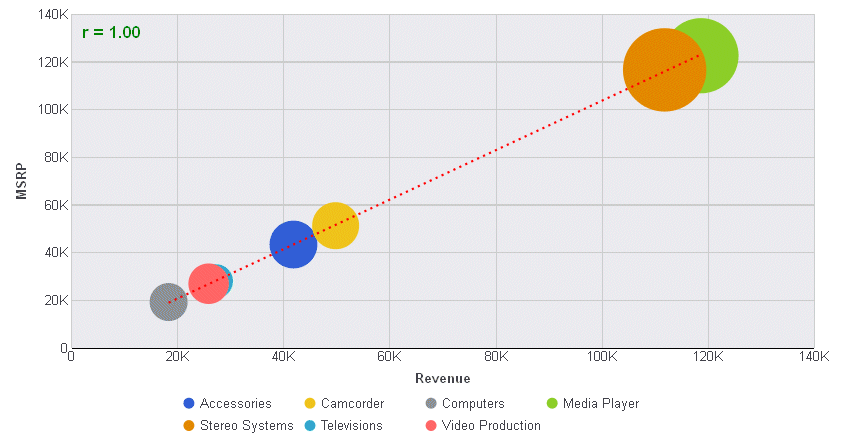Drawing Trendlines in Bubble and Scatter Charts (trendline)
 How to:Draw a Trendline in Bubble and Scatter Charts

The trendline properties draw a trendline and equation label in bubble and scatter charts.

Note: You can also draw a trendline for an individual series, For information, see

The following code shows the properties and default values for the trendline:

```trendline: {
enabled: false,
mode: undefined
line: {
width: 1,
color: 'black',
dash: ''
},
equationLabel: {
visible: false,
font: '8pt Sans-Serif',
color: 'black',
mode: 'equation'
}
},```
 Top of page
Syntax: How to Draw a Trendline in Bubble and Scatter Charts
``` trendline: {
enabled: boolean,
mode: 'string'
line: {
width: number,
color: 'string',
dash: 'string'
},
equationLabel: {
visible: boolean,
font: 'string',
color: 'string',
mode: 'string'
}
}```

where:

enabled: boolean

Enables or disables the trendline. Valid values are:

• true, which enables the trendline.
• false, which disables the trendline. This is the default value.
mode: 'string'

Is string that defines the trendline mode (the type of equation used to generate the trendline). Valid values are:

• 'exponential'.
• 'geometric'.
• 'hyperbolic',
• 'linear'.
• 'logarithmic'.
• 'modExponential'.
• 'modHyperbolic'.
• 'polynomial'.
• 'rational'.
• undefined. This is the default value.
line:

Defines the properties of the trendline.

width: number

Is a number that defines the width of the trendline in pixels. The default value is 1.

color: 'string'

Is a color defined by a color name or numeric specification string that defines the color of the trendline. The default value is 'black'.

dash: 'string'

Is a string that defines the dash style of the trendline. Use a string of numbers that defines the width of a dash followed by the width of the gap between dashes (for example, dash: '1 1' draws a dotted line). The default value is '', which draws a solid line.

equationLabel:

Defines the properties of the equation label.

visible: boolean

Controls the visibility of the equation label. Valid values are:

• true, which shows the equation label.
• false, which does not show the equation label. This is the default value.
font: 'string'

Is a string that defines the size, style, and, typeface of the equation label. The default value is '8pt Sans-Serif'.

color: 'string'

Is a color defined by a color name or numeric specification string. The default value is 'black'.

mode: 'string'

Is a string that defines the equation label mode. Valid values are;

• 'equation' (displays the equation in the format most appropriate for the type of trendline generated. For a linear trendline, the equation displays in slope intercept form). This is the default value.
• 'r' (displays the correlation coefficient).
 Top of page
Example: Drawing Trendlines in Bubble and Scatter Charts

The following request generates a bubble chart and defines a linear trendline that is a red dashed line and has a green equation label that shows the correlation coefficient:

```GRAPH FILE WF_RETAIL_LITE
SUM  REVENUE_US MSRP_US DISCOUNT_US
BY PRODUCT_CATEGORY
ON GRAPH PCHOLD FORMAT JSCHART
ON GRAPH SET LOOKGRAPH BUBBLE
ON GRAPH SET STYLE *
*GRAPH_JS
trendline: {
enabled: true,
mode: 'linear',
line: {width: 2,color: 'red',dash: '2 4'},
equationLabel: {visible: true,font: 'bold 12pt Sans-Serif',color: 'green', mode:'r'}
}
*END
ENDSTYLE
END```

The output is:WebFOCUS